Subsections

# Subdivision curves and surfaces

Subdivision schemes deserve to be widely used because they combine mathematical elegance with an exceptionally simple implementation. For curves, given an arbitrary control polygon, we use the positions of the current vertices to determine the location of the new vertices in a new, refined, control polygon. Generally, each old vertex gives rise to two new vertices. For example, you could place new vertices one-quarter and three-quarters of the way between each adjacent pair of old vertices. Connecting all the new vertices together, in the appropriate order, produces a more refined control polygon. Repeat this process several times and you produce a very good approximation to the uniform quadratic B-spline curve defined by the original set of vertices. In the limit, the refined control polygon becomes this uniform quadratic B-spline curve. The Doo-Sabin subdivision method is the extension of this idea to surfaces. Given the simplicity of the implementation and the fact that you can stop whenever you like, you can see how attractive this method is for computer graphics.

## Mathematical details: curves

Take an arbitrary polygon defined by the sequence of control points: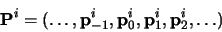Subdivision maps this sequence of control points to a new sequence,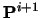by applying subdivision rules. This process doubles9 the number of points, and there is one rule for the odd numbered points and one for the even. For example, the subdivision rules on which the Doo-Sabin method is based are: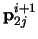=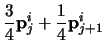(109)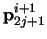=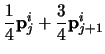(110)

while the subdivision rules on which the Catmull-Clark methods is based are:=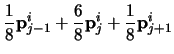(111)=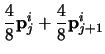(112)

As is the way with much mathematics, we can write it in a more compact, more general, but less obvious, form as: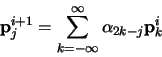(113)

where the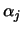are coefficients depending on the subdivision rules. Notes that the index 2k - j alternately selects the even indexedand the odd indexed. So, the two schemes given above, can be compactly described as: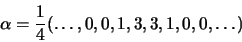(114)

and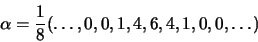(115)

respectively. You will recognise the sequences in parenteses as being two rows from Pascal's triangle.

It would now be constructive for you to draw an arbitrary control polygon and perform a couple of subdivision steps using the first of the two subdivision schemes. Once you feel happy that you understand what is going on, you may like to try the second scheme. For those for whom these two tasks seem simple, you may like to consider what happens if you try to use the previous row from Pascal's triangle (1,2,1) and, for even more excitement, what happens if you try to use the next row (1,5,10,10,5,1). Both produce valid subdivision methods, but you will find that (1,2,1) has a minimal effect on the shape of the control polygon.

## Mathematical details: surfaces

The above subdivision methods can be easily extended from a control polygon to a quadrilateral mesh. This is a mesh where every polygon is a quadrilateral and every vertex is connected to four other vertices.

The Doo-Sabin subdivision method introduces four new vertices in each quadrilateral, and connects up vertices accordingly. The new vertices are blended mixtures of the old vertices in the proportions 9:3:3:1(derived from the tensor product of the univariate case: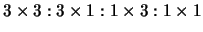). This is illustrated in Figure 2.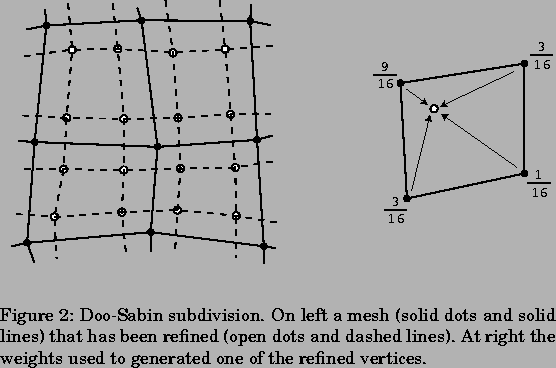Catmull-Clark subdivision is not much more difficult to understand. The only difference here is that is not all of the new vertices are created using the same weights. A vertex is introduced in the centre of each quadrilateral, in the centre of each edge, and near to each old vertex. Each of these three types of vertex has a different set of weights as illustrated in Figure 3.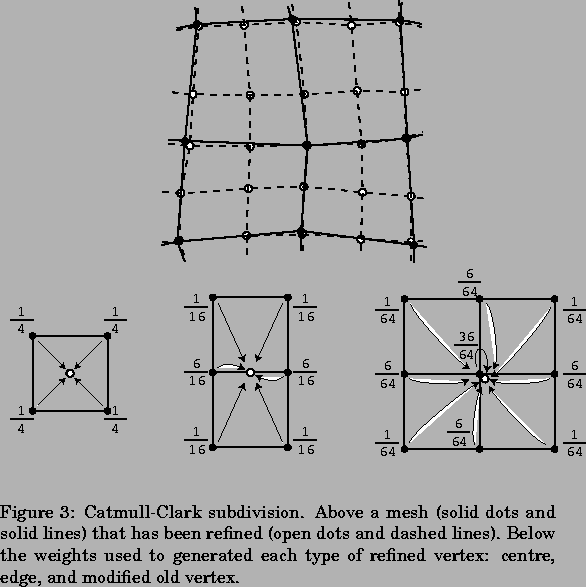This all works beautifully for quadrilateral meshes. Now, suppose we have a quadrilateral mesh that contains extraordinary vertices, in other words a mesh that consists of quadrilaterals but has occasional vertices with other than four immediate neighbours. The Doo-Sabin scheme will still worked quite happily, because every polygon in the mesh is still quadrilateral. However, the Catmull-Clark subdivision scheme depends on every vertex having exactly four neighbours for the generation of the new vertex that is near to the old vertex position (the rightmost case in Figure 3). Catmull and Clark got around this problem by creating a new set of weights, one set of weights for each vertex valence (the valence of vertex is a number of other vertices to which it is connected). Instead of weights of 1/64, 6/64, and 36/64 you have weights of 1/4n2, 3/2n2, and 1-3/2n-1/4n, where nis the valence of the vertex.

However, this is not the only type of mesh with which we can deal. The Doo-Sabin scheme can be easily modified to cope with meshes in which some of the polygons are not quadrilateral, while still maintaining C1-continuity everywhere.

Other schemes, notably the Butterfly, Loop (named after Dr Loop), and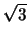schemes handle triangular meshes. Some of these will be discussed in the lectures.